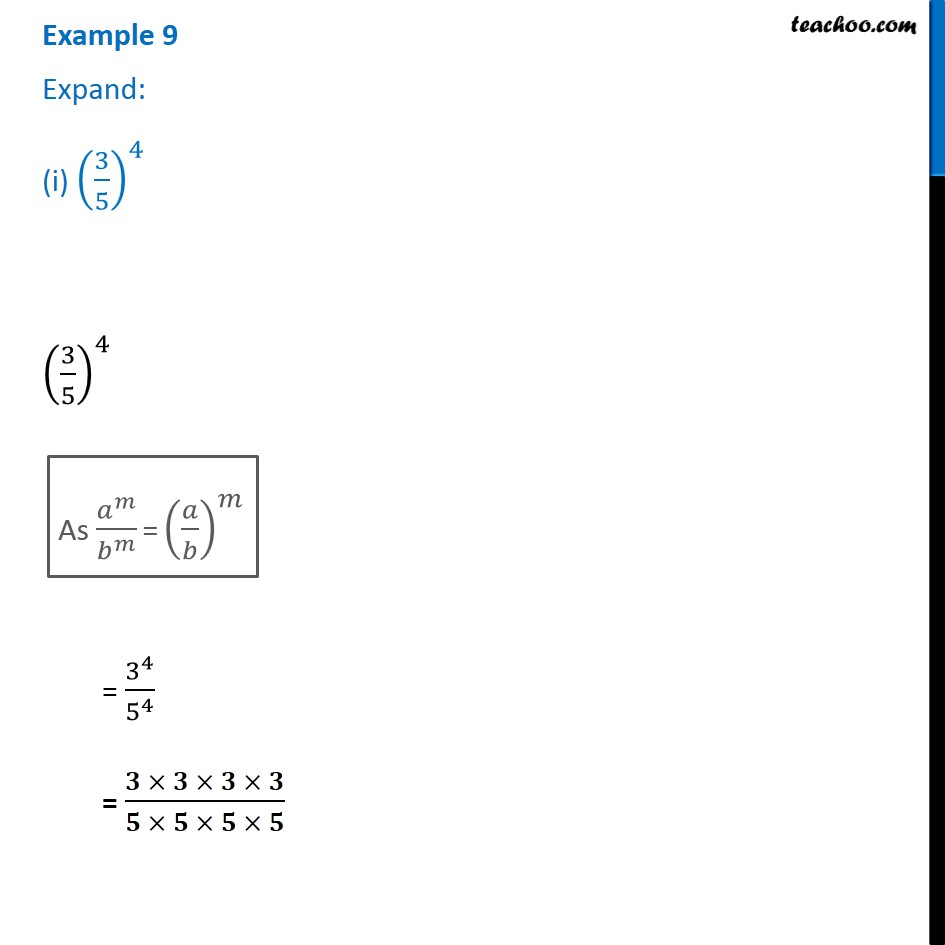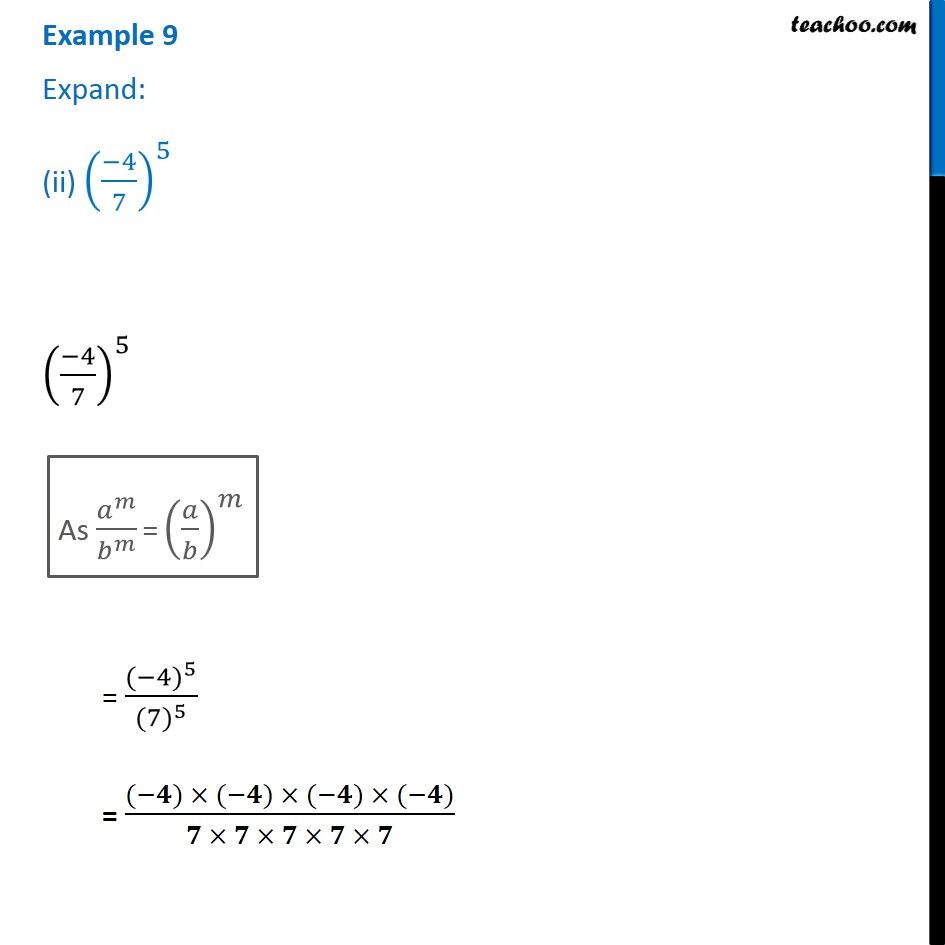1. Chapter 13 Class 7 Exponents and Powers
2. Serial order wise
3. Examples

Transcript

Example 9 Expand: (i) (3/5)^4 (3/5)^4 As 𝑎^𝑚/𝑏^𝑚 = (𝑎/𝑏)^𝑚 (3/5)^4 = 3^4/5^4 = (𝟑 × 𝟑 × 𝟑 × 𝟑)/(𝟓 × 𝟓 × 𝟓 × 𝟓) Example 9 Expand: (ii) ((−4)/7)^5 ((−4)/7)^5 As 𝑎^𝑚/𝑏^𝑚 = (𝑎/𝑏)^𝑚 = 〖(−4)〗^5/〖(7)〗^5 = ((−𝟒) × (−𝟒) × (−𝟒) × (−𝟒))/(𝟕 × 𝟕 × 𝟕 × 𝟕 × 𝟕)

Examples

About the AuthorDavneet Singh
Davneet Singh is a graduate from Indian Institute of Technology, Kanpur. He has been teaching from the past 10 years. He provides courses for Maths and Science at Teachoo.